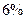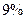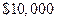Chapter 17, Problem 37P### Principles of Economics 2e

2nd Edition
Steven A. Greenlaw; David Shapiro
ISBN: 9781947172364

#### Solutions

Chapter
Section### Principles of Economics 2e

2nd Edition
Steven A. Greenlaw; David Shapiro
ISBN: 9781947172364
Textbook Problem

# Imagine that a local water company issuedten-year bond at an interest rate ofYou are thinking about buying this bond one year before the end of the ten years, but interest rates are nowa. Given the change in interest rates, would you expect to pay more or less thanfor the bond?b. Calculate what you would actually be willing to pay for this bond.

i

To determine

With the change in the rate of interest, whether more or less than \$10,000 is expected to be paid for the bond is to be determined.

Explanation

As the interest rate rises, then with less face valu...

ii

To determine

To calculate: the face value of the bond.

### Still sussing out bartleby?

Check out a sample textbook solution.

See a sample solution

#### The Solution to Your Study Problems

Bartleby provides explanations to thousands of textbook problems written by our experts, many with advanced degrees!

Get Started